# Midterm 1 Preparation

This chapter contains several sections that will help you prepare for the midterm exam:

# Midterm One: Composition

The first midterm will be composed of fifteen questions covering chapters 1 to 4.

Twelve questions will be algebra questions, and three questions will be word problems.

• Questions 1–4 will be drawn from Chapter 1: Algebra Review and Chapter 2: Linear Equations
• 1.1 Integers
• 1.2 Fractions
• 1.3 Order of Operations
• 1.4 Properties of Algebra
• 1.5 Terms and Definitions
• 2.1 Elementary Linear Equations
• 2.2 Solving Linear Equations
• 2.3 Intermediate Linear Equations
• 2.4 Fractional Linear Equations
• 2.5 Absolute Value Equations
• 2.6 Working with Formulas
• Questions 5–8 will be drawn from Chapter 3: Graphing
• 3.1 Points and Coordinates
• 3.2 Midpoint and Distance Between Points
• 3.3 Slopes and Their Graphs
• 3.4 Graphing Linear Equations
• 3.5 Constructing Linear Equations
• 3.6 Perpendicular and Parallel Lines
• Questions 9–12 will be drawn from Chapter 4: Inequalities
• 4.1 Solve and Graph Linear Inequalities
• 4.2 Compound Inequalities
• 4.3 Linear Absolute Value Inequalities
• 4.4 2D Inequality and Absolute Value Graphs
• Questions 13–15 will be drawn from:

• 1.6 Unit Conversion Word Problems
• 2.7 Variation Word Problems
• 3.7 Numeric Word Problems
• 4.5 Geometric Word Problems

Students will be allowed to use MATQ 1099 Data Booklets for both Midterms and Final Exam.

# Midterm One Review Questions

Questions 1–12 are for review only and will not show up on exams.

1.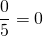(true or false)
2.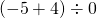3.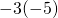4.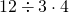5.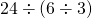6.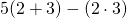7.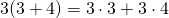(true or false)
8.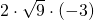9.10.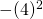11.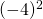12.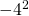Questions similar to 13–20 will show up on exams.

1. Evaluate: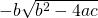if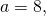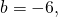and.

## Chapter 2: Linear Equations (Exam Type Questions)

1. Solve for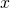in the equation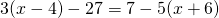.
2. Isolate the variable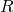in the following equation: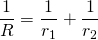3. Solve forin the equation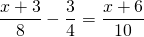## Chapter 3: Graphing (Exam Type Questions)

1. Find the equation of the horizontal line that passes through the point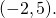2. Find the equation that has a slope of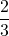and passes through the point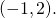3. Find the equation of the line passing through the points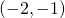and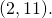4. Graph the relation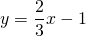.
5. Find the distance between the data points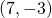and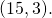6. Find the midpoint between the data pointsand## Chapter 4: Inequalities (Exam Type Questions)

Questions 23–26 are for review only and will not show up on exams.

1. 0 is a whole number (true or false)
2. The elements of the set of integers are elements of the set of rational numbers (true or false)
3. If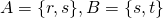and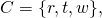then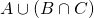equals what?
4.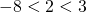(true or false)

Questions similar to 27–37 will show up on exams.

For questions 27 to 30, find each solution set and graph it.

1.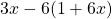>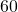2.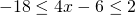3.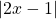>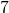4.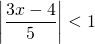5.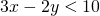6. Graph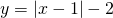.

## Chapter 1–3 Word Problems (Exam Type Questions)

1. Find two numbers such that 6 times the larger number plus 2 times the smaller is 38, and 4 times the larger minus twice the smaller is 12.
2. Karl is going to cut a 36 cm cable into 2 pieces. If the first piece is to be 5 times as long as the second piece, find the length of each piece.
3. Kyra gave her brother Mark a logic question to solve: if she has 14 coins in her pocket worth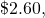and if the coins are only dimes and quarters, how many of each kind of coin does she have?
4. Find two consecutive even integers such that their sum is 10 less than the first integer.
5.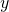varies jointly with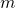and the square of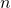and inversely with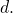If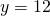when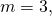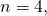and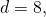find the constant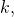then use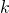to findwhen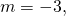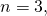and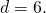<a class=”internal” href=”/intermediatealgebraberg/back-matter/mid-term-1-prep-answer-key/”> Midterm 1: Practice Questions Answer Key